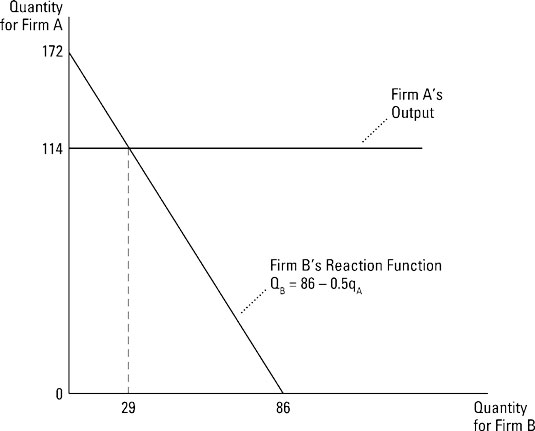##### Microeconomics For Dummies - UK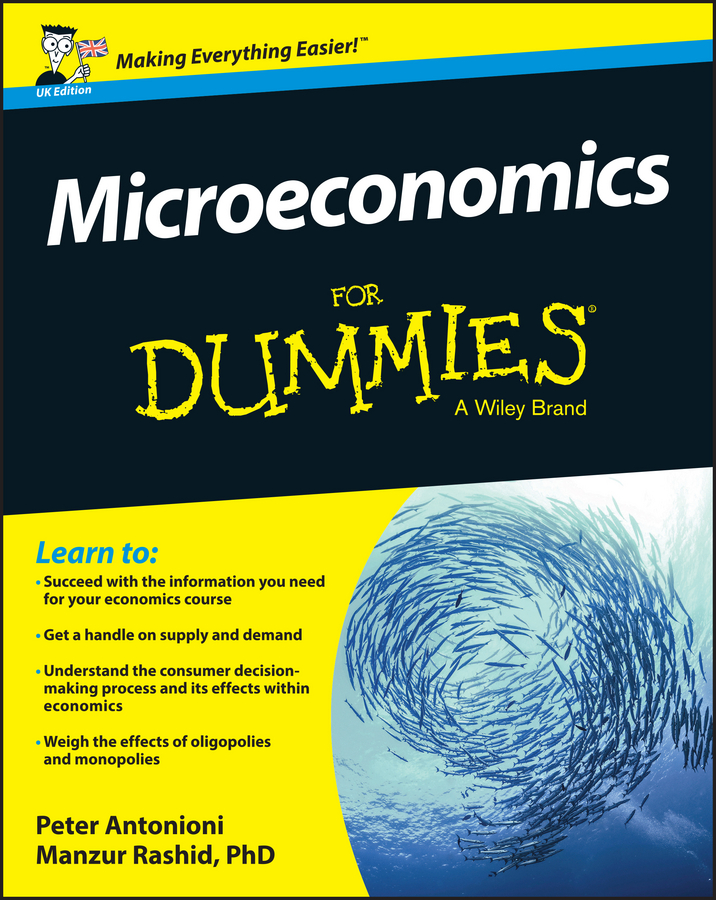Changing the assumptions of how firms react to one another changes the decision-making process. In the Stackelberg model of duopoly, one firm serves as the industry leader. As the industry leader, the firm is able to implement its decision before its rivals.

Thus, if firm A makes its decision first, firm A is the industry leader and firm B reacts to or follows firm A’s decision. However, in making its decision, firm A must anticipate how firm B reacts to that decision.

An example of such leadership may be Microsoft’s dominance in software markets. Although Microsoft can make decisions first, other smaller companies react to Microsoft’s actions when making their own decisions. The actions of these followers, in turn, affect Microsoft.

The primary difference between the Cournot and Stackelberg duopoly models is that firms choose simultaneously in the Cournot model and sequentially in the Stackelberg model.

The market demand curve now faced by the Stackelberg duopolies is: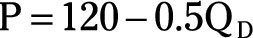where QD is the market quantity demanded and P is the market price in dollars.

Assume that firm A has a constant marginal cost of \$20 and firm B has a constant marginal cost of \$34. Derive the Stackelberg solution with the following steps:

1. Firms A and B provide the entire market quantity demand, QD.

Thus,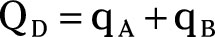2. Substitute qA and qB for QD in the market demand curve to yield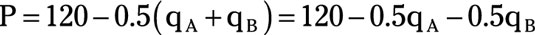3. Because firm B reacts to firm A’s output decision, begin by deriving firm B’s reaction function.

Start by noting that total revenue equals price multiplied by quantity. For price, substitute the equation from Step 2.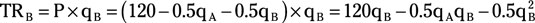4. Firm B’s marginal revenue equals the derivative of total revenue, TRB, with respect to qB.

Treat qA as a constant because firm B can’t change the quantity of output produced by firm A.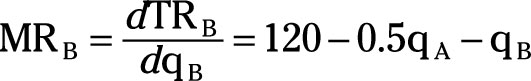5. Firm B maximizes profit by equating its marginal revenue and marginal cost.

Remember that firm B’s marginal cost equals \$34.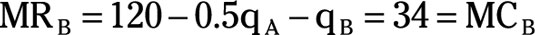6. Rearrange the equation in Step 5 to solve for qB and to get firm B’s reaction function.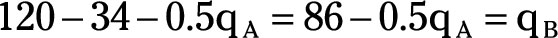For the next step, the demand curve faced by firm A is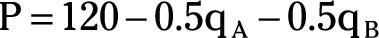7. At this point, substitute firm B’s reaction function into firm A’s demand curve.

This is the critical difference from the Cournot duopoly. By substituting firm B’s reaction function in its decision-making process, firm A is anticipating firm B’s reaction to its output decision.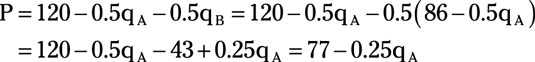8. Firm A’s total revenue, TRA, equals price times quantity.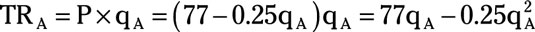9. Firm A’s marginal revenue is the derivative of total revenue taken with respect to qA.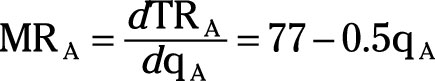10. Firm A determines the profit-maximizing quantity of output by setting marginal revenue equal to marginal cost and solving for qA.

Remember that firm A’s marginal cost is a constant \$20.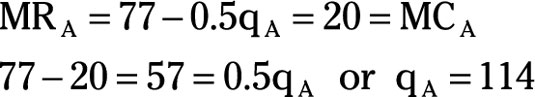11. Substitute qA into firm B’s reaction function from Step 6 to determine qB.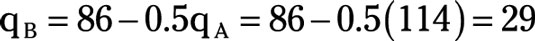Thus, the profit-maximizing Stackelberg duopoly has firm A producing 114 units of output and firm B producing 29 units of output. The illustration shows the Stackelberg duopoly.

Note that firm B has exactly the same reaction function as existed in the Cournot duopoly. On the other hand, firm A doesn’t have a reaction function. Firm A sets it output first, and then firm B reacts to that output. Thus, the horizontal line for firm A at 114 units of output indicates it has set its output before firm B reacts.

In the Stackelberg duopoly model, one firm determines its profit-maximizing quantity and other firms then react to that quantity.

In the Cournot model, firm A simply notes that the market demand is satisfied by the output produced by it and firm B. The two firms make simultaneous decisions. In the Stackelberg model, firm A substitutes an equation to represent how firm B reacts to its production decision. The model reflects sequential decisions.

The simultaneous decision-making associated with the Cournot model leads to different outcomes from the outcomes associated with sequential decisions of the Stackelberg model. The Stackelberg leadership model results in a higher market quantity and lower price for the good as compared to the Cournot model.